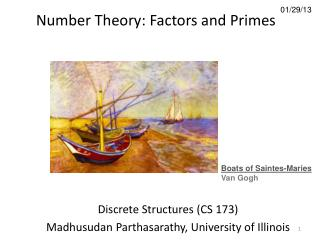DownloadDownload Presentation01/29/13

# 01/29/13

Télécharger la présentation## 01/29/13

- - - - - - - - - - - - - - - - - - - - - - - - - - - E N D - - - - - - - - - - - - - - - - - - - - - - - - - - -
##### Presentation Transcript

1. Number Theory: Factors and Primes 01/29/13 Boats of Saintes-Maries Van Gogh Discrete Structures (CS 173) Madhusudan Parthasarathy, University of Illinois

2. Counting, numbers, 1-1 correspondence

3. Representation of numbers • Unary • Roman • Positional number systems: Decimal, binary

4. ALGORITHMS ZERO (500 AD) al-Khwārizmī : Persian mathematician, astronomer “On the calculation with Hindu numerals”; 825 AD decimal positional number system

5. Natural numbers and integers Natural numbers: closed under addition and multiplication Integers: closed under addition, subtraction, multiplication (but not “division”)

6. Divisibility Suppose and are integers. Then dividesiff for some integer . Example: because “ divides” “” is a factor or divisor of is a multiple of

7. Examples of divisibility • Which of these holds? 4 | 12 11 | -11 4 | 4 -22 | 11 4 | 6 7 | -15 12 | 4 4 | -16 6 | 0 0 | 6 () (, for some integers

8. Proof with divisibility Claim: For any integers , if and b, then . Definition: integer divides integer iff for some integer

9. Proof with divisibility Claim: For any integers , if and , then . Definition: integer divides integer iff for some integer

10. Prime numbers • Definition: an integer is prime if the only positive factors of are and . • Definition: an integer is compositeif it is not prime. • Primalityis in P! [AKS02] • Fundamental Theorem of Arithmetic (aka unique factorization theorem) Every integer can be written as the product of one or more prime factors. Except for the order in which you write the factors, this prime factorizationis unique. 600=2*3*4*5*5

11. GCD • Greatest common divisor (GCD) for natural numbers a and b: is the largest number that divides both and max { n | n N, n | a and n |b}. Defined only if { n | n  N, n | a and n | b} has a maximum. So defined iff at least one of a and b is non-zero. • Product of shared factors of and • Relatively prime: and are relatively prime if they share no common factors, so that

12. LCM • Least common multiplier (LCM): is the smallest number that both and divide lcm(a,b) = min{ p | p N, p >0, a|p and b|p}. • lcm(0,b)=lcm(a,0)=0 by definition.

13. Factor examples gcd(5, 15) = gcd(0, k) = gcd(8, 12) = gcd(8*m, 12*m) = lcm(120, 15) = lcm (6, 8) = Which of these are relatively prime? 6 and 8? 5 and 21? 6 and 33? 3 and 33? Any two prime numbers?

14. Computing the gcd Naïve algorithm: factor a and b and compute gcd… but no one knows how to factor fast! E.g., if and , and

15. Euclidean algorithm for computing gcd is the remainder when is divided by y x

16. Euclidean algorithm for computing gcd is the remainder when is divided by y x

17. Recursive Euclidean Algorithm

18. But why does Euclidean algorithm work? Euclidean algorithm works iff, where

19. Proof of Euclidean algorithm Claim: For any integers , with , if then .

20. Next class • More number theory: congruences • Rationals and reals